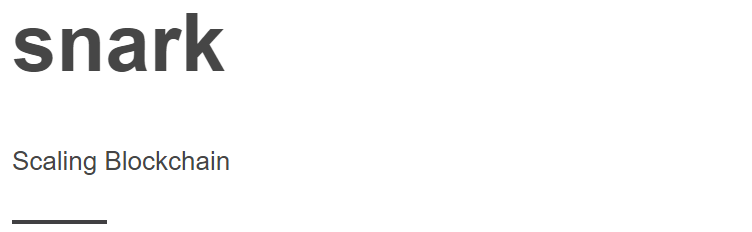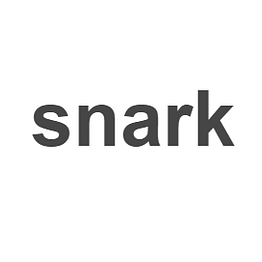# Snark: Token TrilemmaIn our previous analysis we proposed the production cost correlation to token evaluation.

Why does a token need to exist?

To incentivize a provider to provide a service. In the case of Proof of Work, the service is mining (providing hashing power to secure the network). The provider takes an expense x and is paid for with value x + y, with y representing market value markup.

So we have proposed that in a;

• Proof of Work system, production cost is equal to, total energy cost for total hashing power / total tokens generated.
• Proof of Stake system, production cost is equal to, total hosting costs / total tokens generated. …

# Snark: Designing token priceWe have previously discussed the equilibrium value of a token and its impact in a decentralized ecosystem. We settled on Pm < t < Cm, where Pm is the (P)roviders (m)inimum cost and Cm is the (C)onsumers (m)aximum expenditure.

The objective, solve for t within the proposed architecture. We have noticed a current design trend where token price does not take system equilibrium into consideration. We explain this statement shortly.

Solve for Pm

We start with an investigation on current Proof of Work based systems.

We define the following metrics

• Hashing Power (H/s)
• Power Consumption (W)
• Cost per KWh in…

# Snark: ConsensusObjective: Comparison of Consensus protocols

Disclaimer: This is meant to be a comparative benchmark of similar implementations, this is not a comment on specific implementations of consensus algorithms.

Constraints;

• Perfect network
• Transaction backlog near empty
• No transaction validation
• Throughput
• Time To Finality (TTF)
• Data size
• Network awareness
• 100 active balance accounts
`generateAccounts(total) {  accounts = []  for (i = 0; i < total; i++) {    privateKey = crypto.randomBytes(32) …`